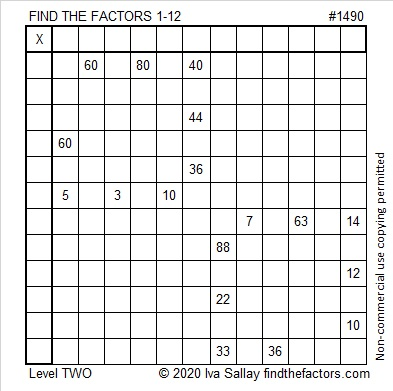# 1490 and Level 2

### Today’s Puzzle:

You can solve this puzzle if you know the basic multiplication and division facts. Just write the numbers from 1 to 12 in both the first column and the top row so the given clues and those factors create a valid multiplication table.### Factors of 1490:

• 1490 is a composite number.
• Prime factorization: 1490 = 2 × 5 × 149.
• 1490 has no exponents greater than 1 in its prime factorization, so √1490 cannot be simplified.
• The exponents in the prime factorization are 1, 1, and 1. Adding one to each exponent and multiplying we get (1 + 1)(1 + 1)(1 + 1) = 2 × 2 × 2 = 8. Therefore 1490 has exactly 8 factors.
• The factors of 1490 are outlined with their factor pair partners in the graphic below.### More Facts about the Number 1490:

1490 is the sum of two squares in two different ways:
31² + 23² = 1490.
37² + 11² = 1490.

1490 is the hypotenuse of FOUR Pythagorean triples:
432-1426-1490, calculated from 31² – 23², 2(31)(23), 31² + 23².
510-1400-1490, which is 10 times (51-140-149).
814-1248-1490, calculated from 2(37)(11), 37² – 11², 37² + 11².
894-1192-1490, which is (3-4-5) times 298.